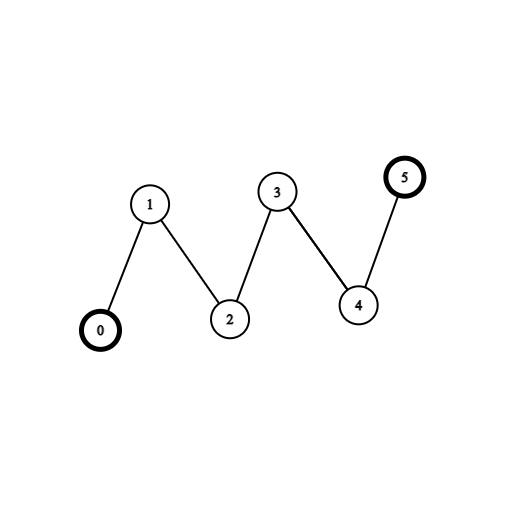2603. Collect Coins in a Tree
Hard
246
7

There exists an undirected and unrooted tree with `n` nodes indexed from `0` to `n - 1`. You are given an integer `n` and a 2D integer array edges of length `n - 1`, where `edges[i] = [ai, bi]` indicates that there is an edge between nodes `ai` and `bi` in the tree. You are also given an array `coins` of size `n` where `coins[i]` can be either `0` or `1`, where `1` indicates the presence of a coin in the vertex `i`.

Initially, you choose to start at any vertex in the tree. Then, you can perform the following operations any number of times:

• Collect all the coins that are at a distance of at most `2` from the current vertex, or
• Move to any adjacent vertex in the tree.

Find the minimum number of edges you need to go through to collect all the coins and go back to the initial vertex.

Note that if you pass an edge several times, you need to count it into the answer several times.

Example 1:```Input: coins = [1,0,0,0,0,1], edges = [[0,1],[1,2],[2,3],[3,4],[4,5]]
Output: 2
Explanation: Start at vertex 2, collect the coin at vertex 0, move to vertex 3, collect the coin at vertex 5 then move back to vertex 2.
```

Example 2:```Input: coins = [0,0,0,1,1,0,0,1], edges = [[0,1],[0,2],[1,3],[1,4],[2,5],[5,6],[5,7]]
Output: 2
Explanation: Start at vertex 0, collect the coins at vertices 4 and 3, move to vertex 2,  collect the coin at vertex 7, then move back to vertex 0.
```

Constraints:

• `n == coins.length`
• `1 <= n <= 3 * 104`
• `0 <= coins[i] <= 1`
• `edges.length == n - 1`
• `edges[i].length == 2`
• `0 <= ai, bi < n`
• `ai != bi`
• `edges` represents a valid tree.
Accepted
3.5K
Submissions
9.3K
Acceptance Rate
37.5%

Seen this question in a real interview before?
1/4
Yes
No

Discussion (0)

Related Topics